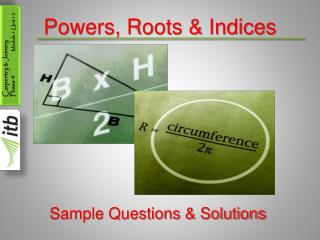DownloadDownload PresentationPowers, Roots & Indices

# Powers, Roots & Indices

Download Presentation## Powers, Roots & Indices

- - - - - - - - - - - - - - - - - - - - - - - - - - - E N D - - - - - - - - - - - - - - - - - - - - - - - - - - -
##### Presentation Transcript

1. Powers, Roots & Indices Sample Questions & Solutions

2. Powers, Roots & Indices • Use repeated multiplication to evaluate the following: (a) 9², (b) 15², (c) 2⁴, (d) 8³, (e) 10³ • Answers: (a) 9 x 9 = 81 (b) 15 x 15 = 225 (c) 2 x 2 x 2 x 2 = 16 (d) 8 x 8 x 8 = 512 (e) 10 x 10 x 10 = 1,000

3. Powers, Roots & Indices • Write the following as a single power: • (a) 5² x 5⁴ • (b) 5³ x 5 • (c) 7 x 7 • (d) 8³ x 8³ • (e) 4² x 4⁶ • (f) 4³ x 4² x 4⁵

4. Powers, Roots & Indices • Answers: • (a) 5² x 5⁴ = 5⁶ (2 + 4 = 6) • (b) 5³ x 5 = 5⁴ (3 + 1 = 4) • (c) 7 x 7 = 7² (1 + 1 = 2) • (d) 8³ x 8³ = 8⁶ (3 + 3 = 6) • (e) 4² x 4⁶ = 4⁸ (2 + 6 = 8) • (f) 4³ x 4² x 4⁵ = 4¹⁰ (3 + 2 + 5 = 10)

5. Powers, Roots & Indices • Express the following as a single power: • 6⁵ 6³ • 7⁶ 7⁵ • 8⁴ 8² • 3⁵ 3 • 4³ 4

6. Powers, Roots & Indices • Answers: • 6⁵ = 6²(5 – 3 = 2) 6³ • 7⁶ = 7(6 – 5 = 1) 7⁵ • 8⁴ = 8²(4 – 2 = 2) 8² • 3⁵ = 3⁴(5 – 1 = 4) 3 • 4³ = 4²( 3 – 1 = 2) 4

7. Powers, Roots & Indices • Write the following as a single power: • (4³)² • (5⁴)³ • (6²)⁴ • (5¹´²)² • (8²´³)³

8. Powers, Roots & Indices • Answers: • (4³)² = 4⁶ (3 x 2 = 6) • (5⁴)³ = 5¹² (4 x 3 = 12) • (6²)⁴ = 6⁸ (2 x 4 = 8) • (5¹´²)² = 5 ( ½ of 2 = 1) • (8²´³)³ = 8² (⅔ of 3 = 2)

9. Powers, Roots & Indices • Calculate the value of the following: • 16¹´² • 36¹´² • 3 x 81¹´² • 5(49)¹´² • 2(25)¹´²

10. Powers, Roots & Indices • Answers: • 16¹´² = √16 = 4 • 36¹´² = √36 = 6 • 3 x 81¹´² = 3 x √81 = 3x 9 = 27 • 5(49)¹´² = 5 x √49 = 5 x 7 = 35 • 2(25)¹´² = 2 x √25 = 2 x 5 = 10

11. Powers, Roots & Indices • Simplify the following: 2⁵ x 2³ 2⁴ 5² x 5⁴ 5³ 8² x 8⁵ 8⁶ 7² x 7⁴ 7³ 3⁷ x 3 3⁵

12. Powers, Roots & Indices • Answers: 2⁵ x 2³ = (5 + 3)- 4 = 8 – 4 = 4 so 2⁴ = 16 2⁴ 5² x 5⁴ = (2 + 4)- 3 = 6 – 3 = 3 so 5³ = 125 5³ 8² x 8⁵ = (2 + 5)- 6 = 7 – 6 = 1 so 8¹ = 8 8⁶ 7² x 7⁴ = (2 + 4)- 3 = 6 – 3 = 3 so 7³ = 343 7³ 3⁷ x 3 = (7 + 1)- 5 = 8 – 5 = 3 so 3³ = 27 3⁵